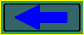Inconsistency of Maxwell's equations and law of Bio-Savar-Laplas is shown.

I. E. Tamm, "Fundamentals of the theory of an electricity", Moscou, Publishing house "Science", 1989.

Let's consider the equation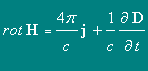(88.5)
Here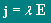(36.5) is a vector of density of a stream of electric ("free") charges
Thus, in electrodynamics, so-called, magnitostatics is the integrated sum of moving charges, and the constant magnetic field is statistical superposition of their magnetic fields.
If in the formula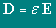(22.4) to define ε = 1 (Vacuum ), that the second member of the right part of the equation 88.5 is possible to copy as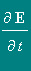.
Intensity of an electrostatic field of a dot charge is expressed by the formula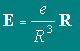(2.1).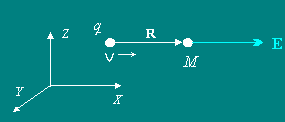It is visible, that at movement of a charge q, in point M, located on an axis of movement, a derivativeis not equal to zero and, according to the formula 88.5,  rot H  also should be nonzero.
However, in the formulation of law of Bio-Savar-Laplas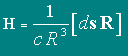(42.2)    the magnetic field, in the same point, should be equal to zero.

This discrepancy of results of two approaches also is offered for discussion.